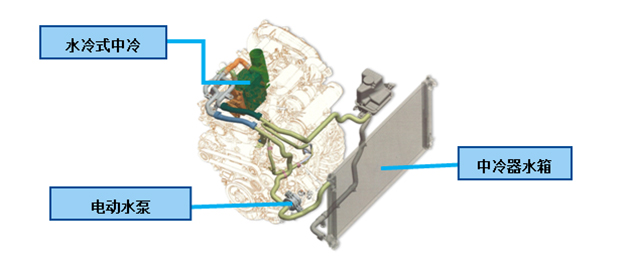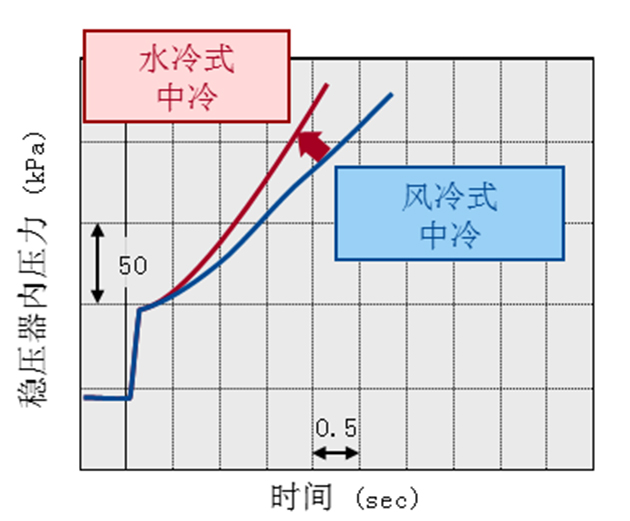## 史上最给力 全新皇冠的水冷技术

2015年08月17日 15:38 来源：www.chextx.com 超过：次关注

面对暑热难耐的桑拿天儿，你会选择怎样的解暑方式？对着风扇一顿猛吹，再猛灌几口冰牙的冰镇饮料，还是直接用水冲凉，然后再品尝几块爽口的西瓜？不管哪种方式都是为了解决酷暑的难耐。人需要解暑，高速运转的发动机同样需要降温。不过今天我们要讲的并不是传统发动机的冷却系统，而是常出现于涡轮增压发动机中的中冷器。中冷器一般人听起来很生涩，它是个什么东东呢？用技术男的口吻告诉你，它就是安装在增压器与发动机进气歧管之间的冷却器，因为位于发动机和增压器之间，所以被称为中间冷却器，简称中冷器。那用隔壁王大妈的话说，它就是个散热的。但显然它不完全算是发动机冷却系统的一部分，那它散的是哪里的热呢？

了解涡轮增压发动机的朋友都知道，涡轮增压发动机比普通发动机拥有更大动力的原因之一，就是其换气效率比自然吸气发动机更高。而进气效率的提高，关键在于涡轮增压，不过当温度非常高的废气驱动涡轮时，会热传导提高进气温度，进气温度升高后，空气密度则会变小氧含量降低（高中物理知识），这样势必会影响发动机的空气压缩效率。本意是要提高进气效率，却碰上“高温”来捣乱，所以中冷器的作用是冷却这部分空气。好了，看来中冷器是涡轮增压的必备套件了。那现在市场上，都有哪些种类的中冷器呢？按照冷却介质的不同，可以分为风冷式和水冷式两种。顾名思义，其中风冷式就是让外界空气流动带走热量，就像前文提到的吹风扇。而水冷式，则是利用循环冷却水，就像前面的冲凉，相比吹风更爽快而且立竿见影。所以二者的冷却效率，不用过多阐述也能高下立判。不过目前市场上，大部分涡轮增压发动机采用的中冷器均为风冷式，原因是它的结构简单、制造成本低，所以尽管不如水冷式的冷却效率高，其应用依旧比较广泛。而与它相反，结构复杂、制造成本较高的水冷式，虽然装机比较少且都是在一些高端机上，但它带来的好处也是多多。

全新皇冠D-4ST 2.0T+发动机采用的正是水冷式中冷器，因为无需很长的管路连接，安装起来特别灵活，让这款发动机的布局更紧凑。另外，冷却效率高，不仅效果好而且用时短。说到这里，小伙伴们一定会问，反复说了半天冷却效果好提升近期压缩效率，有毛用？请看：皇冠D-4ST 2.0T+发动机的173Kw最大功率、350Nm的最大扭矩可并不是盖的。另外，冷却时间短则有利于提升涡轮的响应灵敏度，与世界首次采用的缸盖一体式双涡流技术一起，减轻涡轮迟滞现象，让全新皇冠D-4ST 2.0T+发动机完全可以媲美自然吸气发动机的惬意。

更为重要的是：因为有效防止了涡轮在持续过高温状态下工作，其耐久性和可靠性得到了最大限度的保证，毕竟，谁也不想刚出保修期，就要来个“大手术”换换“器官”吧。其实即便我们不说，相信大家也都知道：丰田，尤其是全新皇冠的可靠性早已达到另人尊敬的地步，毋庸置疑！

全新皇冠的D-4ST 2.0T+ 发动机能有“科技+、动力+、舒适+、可靠+”的四+表现，除了前文介绍过的四双（双喷射、双涡管、双循环、双VVT-i（W））的功不可没以外，水冷式中冷器无疑做出了卓越贡献。但是这也只是这款D-4ST 2.0T+发动机的亮点之一。如果告诉大家这款发动机的最“靠谱”之处到现在还没有说呢，你一定非常惊讶。接下来，我们将具体八一八，全新皇冠的D-4ST如何做到“不烧机油”！

#### 相关文章

﻿
• 快速找车
• 选择品牌
• 选择品牌
• A  奥迪
• A  阿斯顿·马丁
• A  阿尔法·罗密欧
• B  宝沃
• B  布加迪
• B  巴博斯
• B  保时捷
• B  宾利
• B  奔驰
• B  宝马
• B  本田
• B  别克
• B  标致
• B  比亚迪
• B  宝骏
• B  北汽制造
• B  北汽新能源
• B  北汽幻速
• B  北汽威旺
• B  北京汽车
• B  奔腾
• B  北汽绅宝
• B  北汽昌河
• C  长安欧尚
• C  长安
• C  长安凯程
• C  长城
• D  大众
• D  道奇
• D  DS
• D  东南
• D  东风风神
• D  东风风行
• D  东风小康
• D  东风风度
• D  东风
• F  福特
• F  丰田
• F  菲亚特
• F  法拉利
• F  福田
• F  福迪
• F  福汽启腾
• G  观致
• G  广汽传祺
• G  广汽吉奥
• G  GMC
• H  红旗
• H  汉腾汽车
• H  哈弗
• H  哈飞
• H  海格
• H  海马
• H  华颂
• H  黄海
• H  华泰
• H  恒天
• J  捷途
• J  几何汽车
• J  捷达
• J  吉利汽车
• J  捷豹
• J  Jeep
• J  江淮
• J  江铃
• J  金杯
• J  九龙
• J  金旅
• K  凯翼
• K  凯迪拉克
• K  克莱斯勒
• K  科尼塞克
• K  卡威
• K  开瑞
• L  路虎
• L  林肯
• L  劳斯莱斯
• L  兰博基尼
• L  雷克萨斯
• L  铃木
• L  领克
• L  雷诺
• L  理念
• L  力帆
• L  莲花汽车
• L  猎豹
• L  路特斯
• L  陆风
• M  马自达
• M  MG
• M  MINI
• M  玛莎拉蒂
• M  摩根
• M  迈凯轮
• N  纳智捷
• O  欧拉
• O  欧宝
• O  讴歌
• O  欧朗
• Q  奇瑞
• Q  起亚
• Q  启辰
• R  日产
• R  荣威
• R  瑞麒
• S  SERES赛力斯
• S  三菱
• S  斯威汽车
• S  萨博
• S  smart
• S  斯柯达
• S  斯巴鲁
• S  思铭
• S  双龙
• S  上汽大通
• S  双环
• T  特斯拉
• T  腾势
• W  蔚来
• W  沃尔沃
• W  WEY
• W  五菱汽车
• W  五十铃
• W  威兹曼
• W  威麟
• X  现代
• X  雪佛兰
• X  星途
• X  雪铁龙
• X  小鹏汽车
• X  西雅特
• Y  一汽
• Y  英菲尼迪
• Y  英致
• Y  依维柯
• Y  野马汽车
• Y  永源
• Z  众泰
• Z  中华
• Z  中兴
• Z  知豆
• 选择车系
• 选择车系
• 车型对比
• 选择品牌
• 选择品牌
• A  奥迪
• A  阿斯顿·马丁
• A  阿尔法·罗密欧
• B  宝沃
• B  布加迪
• B  巴博斯
• B  保时捷
• B  宾利
• B  奔驰
• B  宝马
• B  本田
• B  别克
• B  标致
• B  比亚迪
• B  宝骏
• B  北汽制造
• B  北汽新能源
• B  北汽幻速
• B  北汽威旺
• B  北京汽车
• B  奔腾
• B  北汽绅宝
• B  北汽昌河
• C  长安欧尚
• C  长安
• C  长安凯程
• C  长城
• D  大众
• D  道奇
• D  DS
• D  东南
• D  东风风神
• D  东风风行
• D  东风小康
• D  东风风度
• D  东风
• F  福特
• F  丰田
• F  菲亚特
• F  法拉利
• F  福田
• F  福迪
• F  福汽启腾
• G  观致
• G  广汽传祺
• G  广汽吉奥
• G  GMC
• H  红旗
• H  汉腾汽车
• H  哈弗
• H  哈飞
• H  海格
• H  海马
• H  华颂
• H  黄海
• H  华泰
• H  恒天
• J  捷途
• J  几何汽车
• J  捷达
• J  吉利汽车
• J  捷豹
• J  Jeep
• J  江淮
• J  江铃
• J  金杯
• J  九龙
• J  金旅
• K  凯翼
• K  凯迪拉克
• K  克莱斯勒
• K  科尼塞克
• K  卡威
• K  开瑞
• L  路虎
• L  林肯
• L  劳斯莱斯
• L  兰博基尼
• L  雷克萨斯
• L  铃木
• L  领克
• L  雷诺
• L  理念
• L  力帆
• L  莲花汽车
• L  猎豹
• L  路特斯
• L  陆风
• M  马自达
• M  MG
• M  MINI
• M  玛莎拉蒂
• M  摩根
• M  迈凯轮
• N  纳智捷
• O  欧拉
• O  欧宝
• O  讴歌
• O  欧朗
• Q  奇瑞
• Q  起亚
• Q  启辰
• R  日产
• R  荣威
• R  瑞麒
• S  SERES赛力斯
• S  三菱
• S  斯威汽车
• S  萨博
• S  smart
• S  斯柯达
• S  斯巴鲁
• S  思铭
• S  双龙
• S  上汽大通
• S  双环
• T  特斯拉
• T  腾势
• W  蔚来
• W  沃尔沃
• W  WEY
• W  五菱汽车
• W  五十铃
• W  威兹曼
• W  威麟
• X  现代
• X  雪佛兰
• X  星途
• X  雪铁龙
• X  小鹏汽车
• X  西雅特
• Y  一汽
• Y  英菲尼迪
• Y  英致
• Y  依维柯
• Y  野马汽车
• Y  永源
• Z  众泰
• Z  中华
• Z  中兴
• Z  知豆
• 选择车系
• 选择车系
• 选择车型
• 选择车型
• 意见反馈